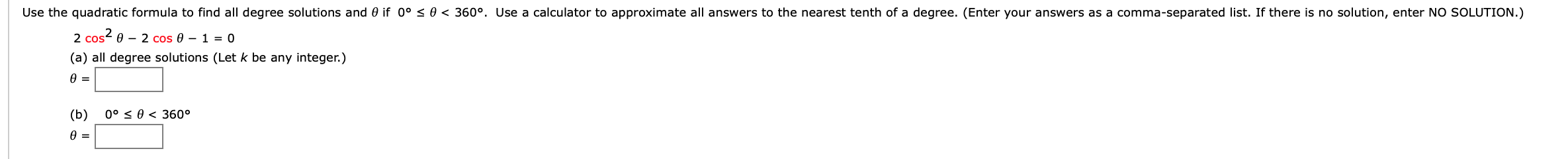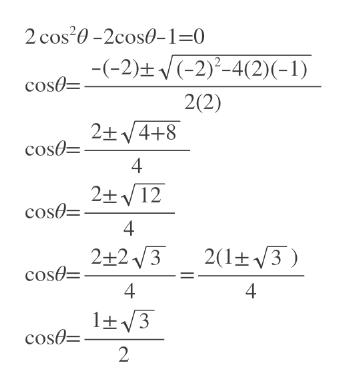# Use the quadratic formula to find all degree solutions and 0 if 0° s 0 < 360°. Use a calculator to approximate all answers to the nearest tenth of a degree. (Enter your answers as a comma-separated list. If there is no solution, enter NO SOLUTION.)2 cos e 2 cos 0 - 1 = 0(a) all degree solutions (Let k be any integer.)(b)0° 0360°

Question
12 viewshelp_outlineImage TranscriptioncloseUse the quadratic formula to find all degree solutions and 0 if 0° s 0 < 360°. Use a calculator to approximate all answers to the nearest tenth of a degree. (Enter your answers as a comma-separated list. If there is no solution, enter NO SOLUTION.) 2 cos e 2 cos 0 - 1 = 0 (a) all degree solutions (Let k be any integer.) (b) 0° 0 360° fullscreen
check_circle

Step 1

First we solve for cos theta using quadratic formula.

cos theta is between -1 to 1.

So cos theta = (1+sqrt(3))/2= 1.366 is not possible

cos theta = (1-sqrt(3))/2= -0.366help_outlineImage Transcriptionclose2 cos20-2cos0-1=0 -(-2)t-2)-4(2)(-1) cosa 2(2) 2+4+8 cosO 4 2+ 12 cos= 4 2(1+3 2+23 cose 4 4 1t3 cos 2 fullscreen
Step 2

Then we find theta using cos inverse.

Answ...

### Want to see the full answer?

See Solution

#### Want to see this answer and more?

Solutions are written by subject experts who are available 24/7. Questions are typically answered within 1 hour.*

See Solution
*Response times may vary by subject and question.
Tagged in

### Trigonometry